# KVAR Energy Controller Report

Ben Wiener, Physics Department, Brandeis University.
Kevan Hashemi, Physics Department, Brandeis University.
www.bndhep.net

## Contents

Introduction
Theory
Experimentation
Studies from Other Labs
Conclusion

## Introduction

The KVAR Energy Controller (or KEC) is a device made by the KVAR Corporation. The company claims that the KEC reduces overall energy costs for a home or business by 6% to 25%. Information about the KEC can be found on their website's how it works page. We were given several studies that KVAR referenced as proof of their product's efficacy. These studies shared their results, but did not describe their testing apparatus or procedure. We tested the KEC ourselves, to determine whether or not the device could reduce a residential electricity bill.

## Theory

The power factor of an AC circuit is the real power it consumes divided by the apparent power it consumes. The real power can never exceed the apparent power. Apparent power is the RMS voltage applied to the circuit multiplied by the RMS current flowing into it. When the peak of the applied voltage cycle does not coincide with the peak of the consumed current cycle, we say the current is out of phase with the voltage. We measure the phase difference between the current and voltage in the following manner. First we measure the time delay between the peak in the current cycle and the peak in the voltage cycle (see here for example cycles). We divide this time delay by the period of the AC cycles, which is 16.7 ms for 60-Hz and 20 ms for 50-Hz. We multiply this fraction by 360° and we end up with an angle that represents the phase difference. It turns out that the power factor of the circuit is equal to the cosine of the phase difference.

When phase difference is negative, we say that current is lagging the voltage, and the circuit itself we describe as inductive. Motors and transformers are examples of inductive circuits. When the phase difference is positive, we say that current is leading the voltage, and the circuit itself is capacitive. Capacitors are the only commonly-occuring examples of capacitive circuits. The power factors of common inductive and capacitive circuits are between zero and one. No circuit can have a power factor less than −1 or greater than +1.

In nearly all cases, the load on a home's electrical supply is inductive due to the presence of motors and transformers. The idea of power factor correction is to bring current and voltage back into phase by counteracting an inductive load by adding a capacitor. The correct capacitor will minimize the current consumed by the home at the same time as bringing the current and voltage exactly into phase, and leaving the real power unchanged.

The KVAR corporation claims that their KEC device reduces a home's energy bill by correcting its power factor to one.

## Experimentation

We decided to first look into the possibility that homes are charged for their apparent power consumption and not just for their real power as one might assume. We obtained two typical home watt-hour meters. These were a Westinghouse CL-200 meter which we called Meter 1 and a General Electric CL-200 meter which we called Meter 2. These meters are designed to accept power directly from the wires that come to a house or business, not to plug into a wall outlet. The way power comes into most houses is in the form of a ground line and two 120V AC lines which are 180 degrees out of phase. We had to find a way to replicate this setup from a single phase, 120V wall outlet. The following schematic shows the setup we used. A 115V isolation transformer allowed us to get the voltages we needed.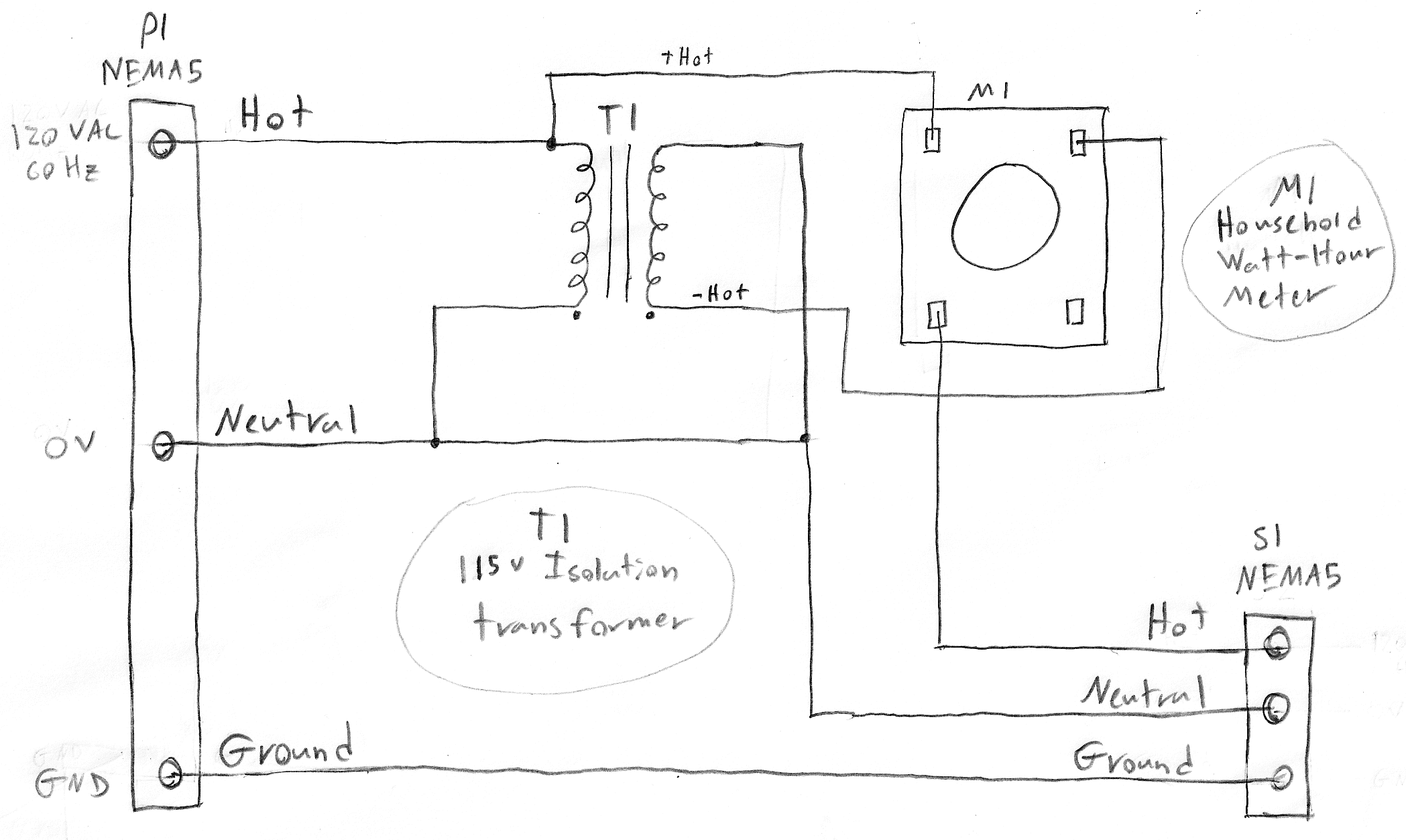Schematic for operation of home watt-hour meter from the single phase, 120V wall outlet on the left. The socket on the right is the output which is also a single phase, 120V wall outlet.

We attached several inductive loads and recorded how far the wheel in the watt-hour meter turned in 3 minutes. We added capacitance in parallel to cancel out the inductance of the load and repeated the measurement. We increased the capacitance slowly until the power factor reached one and began to decrease again. Below is a graph showing our results. Each point shows the percent deviation of power at each capacitance from the mean power level in that trial. No point deviates by more than 1%, indicating that the meter measures only real power.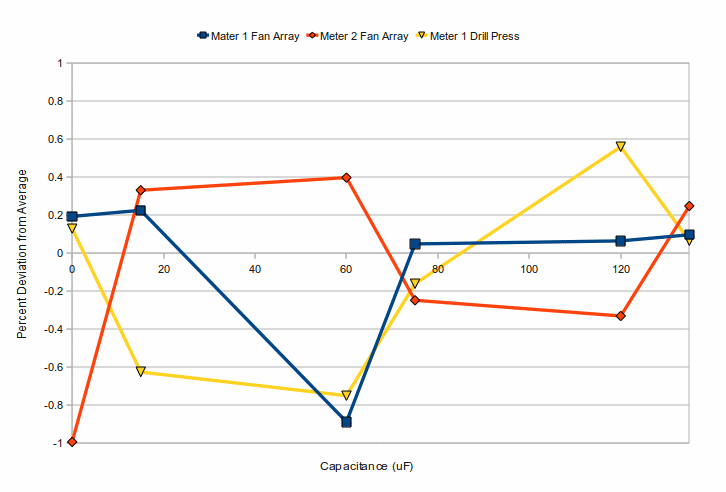We saw no correlation between power factor and real power as measured by the watt-hour meters. This shows that simply reducing the apparent power used by a load without changing the real power consumed, does not decrease the power that an electric company would measure. Further testing with a Kill A Watt digital power meter allowed us to see clearly what was going on. We used it to measure the power consumed and current drawn by a half-horsepower A.O. Smith AC motor as we added capacitance in parallel. Below is a graph of real power, apparent power, and current used by the load.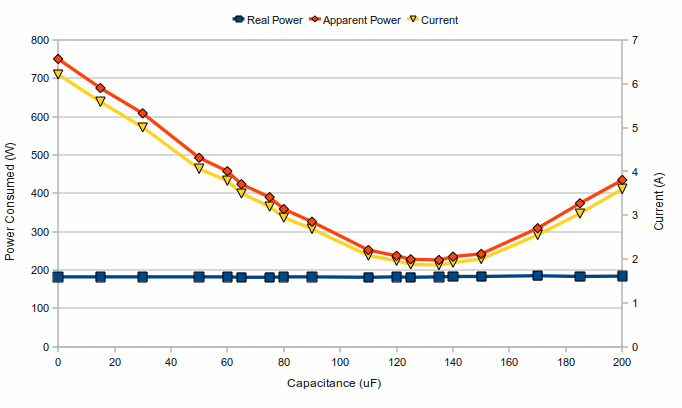As we increased capacitance, the phase between the current and voltage was reduced and the load used less apparent power and drew less current. All the while, real power was unchanged. As current and voltage came back into phase, the apparent power became almost equal to the real power and the power factor neared one. However, according to the Kill A Watt, the apparent power never actually reached the real power. This suggested that we had somehow failed in removing the phase difference between current and voltage. We used an oscilloscope to look at the the actual current and voltage waveforms. Below are the waveforms before and after power factor correction respectively.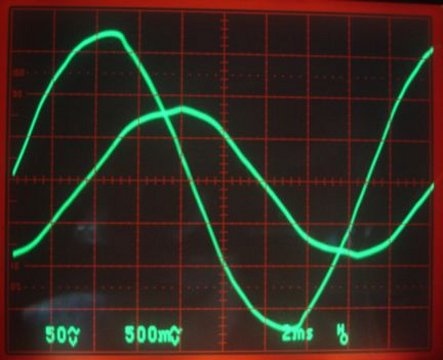Load: 1/2 hp AC motor. Larger trace: Voltage (50 V/Div). Smaller Trace: Current (500 mV/Div across 1Ω = 0.5 A/div). The phase difference is 360° × −2.5 ms offest / 16.7 ms period ≈ −54°, so the current is lagging the voltage, and the load is inductive. The power factor is 0.59.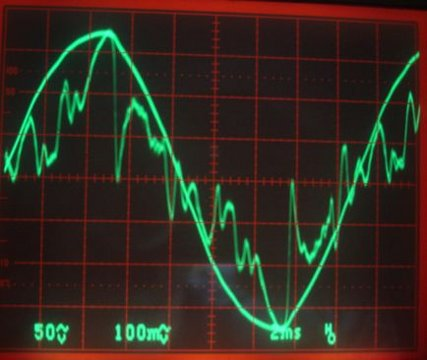Load: 1/2 hp AC motor with 135 μF in parallel. Smooth trace: Voltage (50 V/Div). Distorted Trace: Current (100 mV/Div across 1Ω = 0.1 A/div). The phase difference is 360° × 0 ms offest / 16.7 ms period ≈ 0°, so the power factor is 1.0.

Though the current waveform after power factor correction is severely distorted, it is possible to see that the phase is very close to zero. It may be that the distortion of the current waveform deceived the Kill A Watt, which is designed to deal with sinusoidal waves. Still, it is clear that power factor correction had no effect on the real power consumption, as measured by the same type of meter that an electric company would use.

Even though the watt-hour meter did not register anything but real power, the KEC still has a chance to prove its worth. As we saw in our measurements with the Kill A Watt, the KEC does reduce the current in some parts of a circuit. Lower current means less power dissipated in wires. It is easy to imagine a situation where an inductive load sits far away from the house watt-hour meter. In an extreme case, there might be very long wires and significant energy loss in those wires. However, between the KEC and the load, the current is still present. If the capacitor and inductor are close together and have long wires leading to them, power savings due to reduced dissipation in wires could be significant. However, unless the KEC sits near that device (not near the meter and breaker box), the power savings would be negligible. If the KEC were positioned next to the house watt-hour meter, the excess current will be eliminated only in the short distance between the meter and the KEC, not in the long cable between the KEC and the load. This means that the KEC cannot be both central and effective. If the KEC is to provide power savings in a house, the inductive loads in the house must all be located together in the same place with the KEC.

We simulated long cables with power resistors. We placed our simulated long cables in two ways: between the meter and the KEC (so that the KEC is 'near' the load) and between the KEC and the load (so that the KEC is 'near' the breaker box and meter). Below are the schematics for the two setups (in the same order).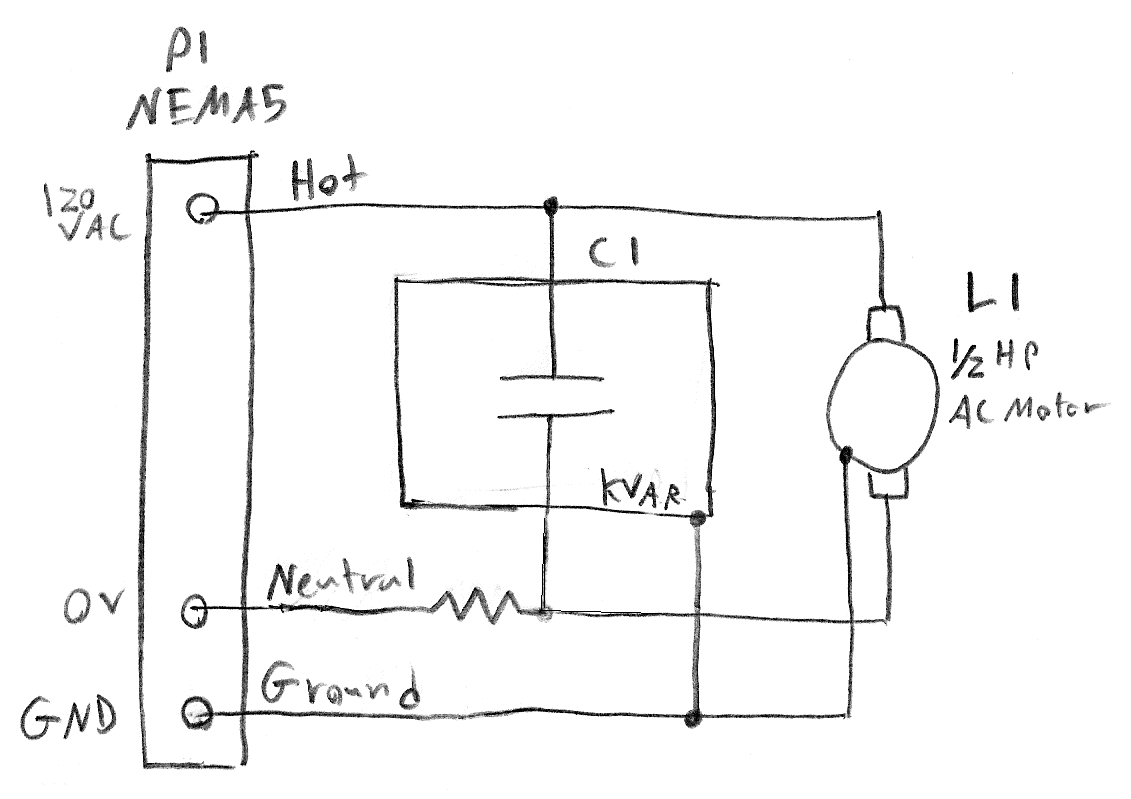Schematics for simulating lengths of wire between the meter and the KEC (left) and between the KEC and the load (right). We varied the resistance of the resistor in each schematic.

We incremented the resistances from 0Ω to 3.5Ω and measured the real power with the long simulated cable between the meter and the KEC and between the KEC and the load.The above graph shows that, if the KEC is near the breaker box and meter, it has no observable effect the real power consumed. If it is installed near the inductive load, it can have an effect which depends on the resistance of the cable between the load and the meter. Consider a situation where our AC motor sits 50 meters from the energy meter with its own KEC nearby and is wired with Romex-12 copper wire. The total 100 m of cable has resistance 0.52 Ω. Assuming the KEC corrects the power factor perfectly, it will reduce the current flowing in the cable from 6.2 A to 1.8 A (see graph). Power dissipated in the cable will drop from 20 W (6.2 A × 6.2 A × 0.52 Ω) to 2 W (1.8 A × 1.8 A × 0.52 Ω), for a savings of 18 W. If electricity costs about \$0.15 per KWh and the motor runs for 10 hours per day, the KEC installed next to the motor would save us about \$11 a year.

We had assumed that the KEC was capable of reading the inductance of the load and changing its own capacitance to match. We measured its capacitance with a multimeter and read a capacitance of about 55μF. We looked at the KEC's effect on the apparent power of two different inductive loads. In both cases, the effect was the same as would be achieved by connecting a 55μF capacitor. Finally, we subjected the KEC and a 58μF capacitor to a square wave to observe them charge and discharge. Below are the oscilloscope traces of the KEC and the capacitor.Oscilloscope traces of voltage across the KEC, capacitor, and voltage source. The KEC and capacitor were not tested simultaneously. The KEC's waveform was saved and put onto the capacitor's graph for comparison.

The traces are nearly identical. The KEC charges and discharges slightly faster due to its lower capacitance. The KEC is a capacitor. There is no internal switching device to change its capacitance.

## Studies from Other Labs

As I mentioned above, we know of several studies about the KEC. There is one study from NASA . It claims to have found energy savings but gives almost no detail about how the experiment was performed. Another study from the University of Santa Clara also claims to have found power savings. They have a schematic which shows a setup similar to our own but without the simulated wires. Their results agree with ours. They found a decrease in current and in apparent power. However, they never mention real power, nor do they address the issue of power company energy measurement. We also found a study from Hodges Electrical Enterprises, Inc. which contained some measurements that we don't understand. They ran a motor connected to an energy meter and timed how long it took for the meter to measure one KWh. However, they don't seem to have used an precise enough meter. The study says that the energy meter starts at 47 KWh and goes to 48 KWh in 4 hours, 31 minutes, and 54 seconds while consuming 196 watts. That only amounts to about 0.88 KWh but they treat it like the motor used one KWh in that time. Finally, we looked at a report by SMT Engineering LLC. They were given a KEC demo box to do tests on. When the opened it up, they found a length of wire between the energy meter and the KEC. They estimate the resistance of this wire to be 0.7Ω. They did not measure the resistance. Their findings agree with ours. However, they fail to discuss the significance wire's placement.

## Conclusion

The placement of the KEC is what determines its effectiveness. Home watt-hour meters measure only real power. Only the extra real power dissipated in wires due to excess current going to inductive loads that can eliminated by the KEC. Placing a KEC at a home's breaker box will not reduce real power consumption. If the KEC is next to an inductive load, it can be effective. According to our above example, adding a KEC to a half-horsepower motor with 100 meters of Romex-12 cable between it and the breaker box will reduce power consumption by 20W as long as the KEC is next to the motor. If there are two such loads in different places, each at the end of its own long cable, the two loads would each require a separate KEC. Also, according to our tests, the KEC is simply a capacitor in a box, not an adaptive power factor correction device. A single capacitor does not justify the going price of several hundred dollars. We were able to buy similarly sized capacitors from Digi-Key for about \$20.